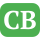CppBuzz.com

# Accessing base Data members from derived class object

```I want to display the data member of Base from derived class object.
when dprint() is called length, width, height, volume of cube displayed
but when print() is called area is correctly displayed but length & width of cube is displayed and not of rectangle class.

#include<iostream>
using namespace std;

class Rectangle
{
protected:
int length,width,area;
public:
void getdata()
{
cout<<"enter data\n";
cin>>length>>width;
area=length*width;
}
void print()
{
cout<<"Rectangle "<<length   <<"    "<<width<<"   "<<area<<"\n";

}
};

class cube : public Rectangle
{
int height,volume;
public:
void getd()
{
cout<<"enter height, length,width";
cin>>height>>length>>width;
volume=length*height*width;
}
void dprint()
{
cout<<"Cube   "<<length<<"    "<<width<<"    "<<height<<"  "<<volume;
}
};

int main()
{
cube c;
c.getdata();
c.getd();
c.print();
c.dprint();
return 0;
}```

Posted by KiranRaj 2019-02-26 08:43:45

```/* Hi KiranRaj, you were doing few things wrong in your code. Please check this code & then run. This is the write way to do what you  are trying to do */

#include<iostream>
using namespace std;

class Rectangle
{
protected:
int length,width,area;
public:
void getdata()
{
cout<<"enter data :
";
cin>>length>>width;
}
void print()
{
area=length*width;
cout<<"Rectangle - "<<length   <<"  *  "<<width<<" =  "<<area<<"
";

}
};

class cube : public Rectangle
{
int height,volume;
public:
void getdata()
{
cout<<"enter height, length,width : ";
cin>>height>>length>>width;
}
void dprint()
{
volume=length*height*width;
cout<<"Cube  - "<<length<<"  *  "<<width<<"  *  "<<height<<" = "<<volume;
}
};

int main()
{
cube c;
c.getdata();
c.print();
c.dprint();
return 0;
}```Admin posted 2019-02-26 22:46:11